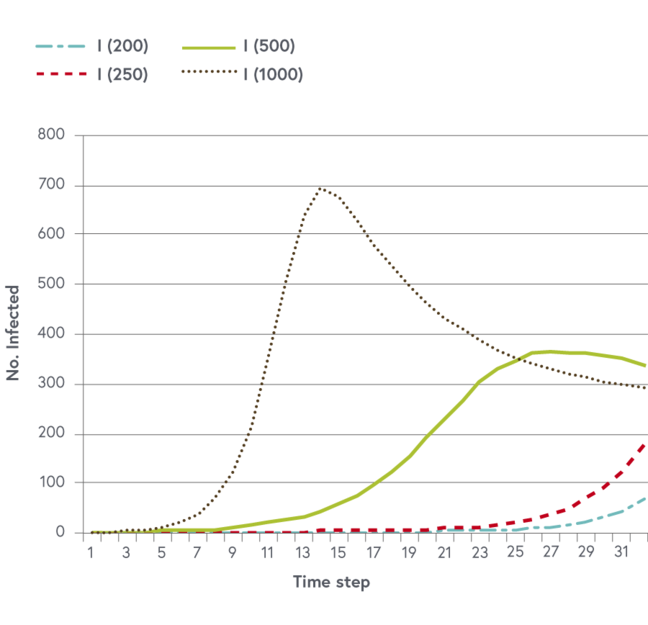2.14

# In depth: disease transmission mathematical model properties

In the last steps we have seen that populations are very dynamic and that we can approach their size and growth with different equations and calculations. In this step, we deepen this understanding and discover that, for example, the spread of an illness can be interrupted by vaccinating a certain amount of individuals.

#### Steady state conditions and the basic reproductive ratio

In many situations, we lack sufficient data to explore the model behavior but we can investigate important model properties considering the differential equations. They describe changing quantities, and we can study their equilibrium conditions by setting them to zero. For example, we may investigate equilibrium conditions of the infectious compartment $I$.

$$\frac{dI}{dt} = \beta IS - \mu I - \delta I = 0 \color{red}{→} \beta IS = (\mu + \delta)I \color{red}{→} S = \frac{(\mu + \delta)}{\beta}$$

Equation one

The compartment $I$ reaches an endemic equilibrium when the number of susceptible reaches a particular size, which in the above example is $S = (\frac{0.05+0.2}{0.001}) = 250$. Another interpretation of this steady state condition is that the number of susceptible individuals at the start of the epidemic must be greater than a certain fixed amount if the infection is to spread and persist in the population. In image one simulations of the infectious compartment are presented with different sizes of susceptible populations at onset. We see that below 250 susceptible individuals the transmission will not take off.Image one: number of infectious individuals with varying numbers of susceptibles (200, 250, 500 and 1000)

The basic reproductive ratio (BRR or $R_0$) is defined as the average number of secondary infections produced when one infected individual is introduced into a host population where every host is susceptible. The $R_0$ describes the potential spread of an infectious disease and its regulation in a host population. If the $R_0$ is above 1 the disease keeps spreading. If $R_0$ is below 1 the disease will not be maintained but will become extinct. We may start again from equation one describing the change of infectious individuals.

$$\frac{dI}{dt} = \beta IS - \mu I - \delta I \color{red}{→} \beta S ≥ (\mu + \delta) \color{red}{→} \frac{\beta S}{\mu + \delta} ≥ 1 = R_e$$

Equation two

The $R_0$ is greater than 1, and hence the disease keeps spreading if $\beta S$ is greater than $(\mu I + \delta)$. Its ratio is called the effective reproductive ratio ($R_e$) and changes with the number of susceptible hosts. At the beginning of an epidemic, all individuals are susceptible and $S = N$. The basic reproductive ratio is then expressed as

$$R_0 = \frac{\beta N}{\mu + \delta} \color{red}{→} N = R_0 \frac{(\mu + \delta)}{\beta}.$$

Equation three

If we solve this for $N$ we again obtain the above-mentioned threshold population size needed for an epidemic to start. A very useful application of the BRR is to explore the minimum vaccination coverage necessary to eradicate a disease. If vaccination coverage is $p$, the number of remaining susceptible hosts becomes $(1 - p)N$. The critical vaccination level can be derived as follows

$$R_0 (1 - p_c) = \frac{\beta N (1 - p_c)}{(\mu + \delta)} = 1 \color{red}{→} R_0 (1 - p_c) = 1$$ $$p_c = 1 - \frac{1}{R_0}.$$

Equation four